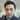# Java - How to override hashCode

February 06, 2017

In Effective Java(2/E), it describes hashCode method always should be overridden when equals method overridden.

Then why??

Let’s have a look at this example case.

``````public class Item {
private final int id;
private final String name;

public Item(int id, String name) {
this.id = id;
this.name = name;
}

@Override
public boolean equals(Object obj) {
if (obj == this) {
return true;
}

if (!(obj instanceof Item)) {
return false;
}

Item item = (Item) obj;

return this.id == item.id
&& this.name.equals(item.name);
}
}``````

When we use this class with HashMap like below,

``````Map<Item, String> map = new HashMap<>();
map.put(new Item(1, "item1"), "result1");

System.out.println(map.get(new Item(1, "item1")));``````

We might expect “result1” as the result, but actual is null. Because Item class does not have hashCode overridden, so two Item instances above have different hash code.

Object.hashCode should follow 3 general contract below: (Java API Specification 7)

• Whenever it is invoked on the same object more than once during an execution of a Java application, the hashCode method must consistently return the same integer, provided no information used in equals comparisons on the object is modified. This integer need not remain consistent from one execution of an application to another execution of the same application.
• If two objects are equal according to the equals(Object) method, then calling the hashCode method on each of the two objects must produce the same integer result.
• It is not required that if two objects are unequal according to the equals(java.lang.Object) method, then calling the hashCode method on each of the two objects must produce distinct integer results. However, the programmer should be aware that producing distinct integer results for unequal objects may improve the performance of hash tables.

To follow contract above, Let’s override hashCode for Item class.

``````@Override
public int hashCode() {
return 10;
}``````

This hashCode follows general contract above, but this is not the right way. Because every Item instances will have the same hash code, and that means the HashMap will store every instances to the same bucket, so performance of the HashMap will be extremely poor.

To design hashCode method efficiently, refer to code below.

``````@Override
public int hashCode() {

int result = 17;

// Include a hash for each field.

// Primatives

// 1 bit   » 32-bit
result = 31 * result + (booleanField ? 1 : 0);

// 8 bits  » 32-bit
result = 31 * result + byteField;
// 16 bits » 32-bit
result = 31 * result + charField;
result = 31 * result + shortField;
// 32 bits » 32-bit
result = 31 * result + intField;

// 64 bits » 32-bit
result = 31 * result + (int)(longField ^ (longField >>> 32));

// 32 bits » 32-bit
result = 31 * result + Float.floatToIntBits(floatField);

// 64 bits (double) » 64-bit (long) » 32-bit (int)
long doubleFieldBits = Double.doubleToLongBits(doubleField);
result = 31 * result +
(int)(doubleFieldBits ^ (doubleFieldBits >>> 32));

// Objects

// var bits » 32-bit
result = 31 * result + Arrays.hashCode(arrayField);

// var bits » 32-bit
result = 31 * result + referenceField.hashCode();
result = 31 * result +
(nullableReferenceField == null
? 0
: nullableReferenceField.hashCode());

return result;

}``````

About constant in the code like 17 and 31, first 17 should be a number which is not 0(zero), and in case of 31, it should be prime number. Please refer to effective java or google search for further mathematical details.

Finally, let’s write proper hashCode method for the Item class. There are 2 ways to properly override.

Traditional way which was introduced in Effective Java,

``````public class Item {
private final int id;
private final String name;

public Item(int id, String name) {
this.id = id;
this.name = name;
}

@Override
public boolean equals(Object obj) {
if (obj == this) {
return true;
}

if (!(obj instanceof Item)) {
return false;
}

Item item = (Item) obj;

return this.id == item.id
&& this.name.equals(item.name);
}

@Override
public int hashCode() {
int result = 17;

result = 31 * result + id;
result = 31 * result + (name == null ? 0 : name.hashCode());

return result;
}
}``````

Another way for Java 7 or above, Objects class just simply can deal with it.
``````public class Item {
private final int id;
private final String name;

public Item(int id, String name) {
this.id = id;
this.name = name;
}

@Override
public boolean equals(Object obj) {
if (obj == this) {
return true;
}

if (!(obj instanceof Item)) {
return false;
}

Item item = (Item) obj;

return this.id == item.id
&& this.name.equals(item.name);
}

@Override
public int hashCode() {
return Objects.hash(id, name);
}
}``````Written by Hakjoon Sim who lives Seoul. Like programming and doing nothing.